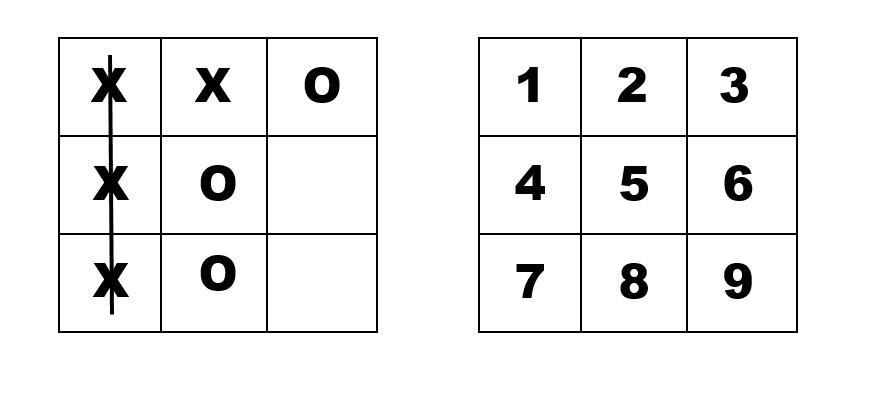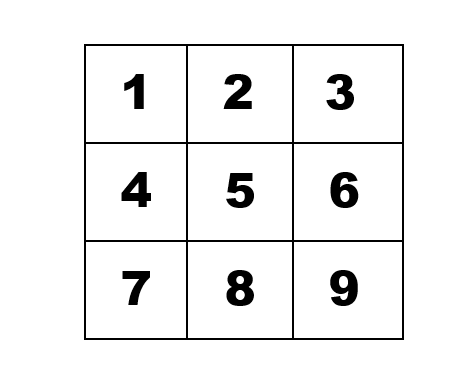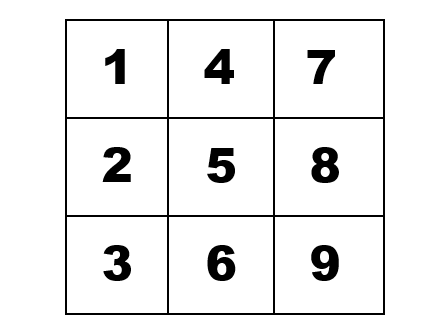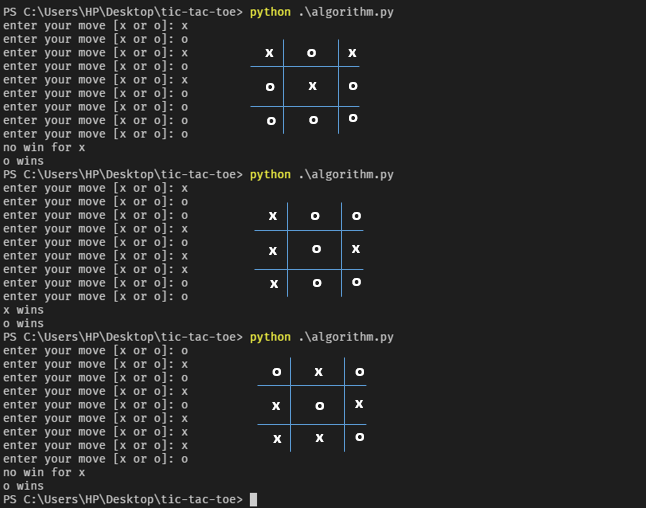×

Search anything:

# Algorithm to check win in tic-tac-toe

#### Algorithms game theoryGet this book -> Problems on Array: For Interviews and Competitive Programming

In this article, we have presented an algorithm to check win and loss for a given Tic Tac Toe configuration.

NOTE: this algorithm assumes that the game data is passed in the following format

``````data = [
# the lists are rows while the individual dictionaries are tiles (the move made and the tile number)
[
{'move': 'x', 'number': 1},
{'move': 'o', 'number': 2},
{'move': 'x', 'number': 3}
],
[
{'move': 'x', 'number': 4},
{'move': 'o', 'number': 5},
{'move': 'o', 'number': 6}
],
[
{'move': 'x', 'number': 7},
{'move': 'x', 'number': 8},
{'move': 'x', 'number': 9}
]
]
``````

Why the above format? take a look at the image belowFrom the above image, we can see that there is one big block which is like the container, we have rows (the blocks that run horizontally from left to right) and columns (blocks that run vertically from up to down) and each row has 3 smaller blocks or tiles.
In our code above, we have the same structure:

1. We have one list (named as data) which is like the container
2. The other 3 lists inside the "container list" stand for the 3 rows.
3. Inside each row we have a tile, which is represented as python dictionaries. Why? This is because we need to keep track of a move and the tile number the move was made on.

Now, lets talk about the implementation of the algorithm
For this example, we will be taking X as our "winning" move (to make things easier).

### STEP 1: What counts as a win?

1. if the same move spans across (horizontally) on one row, that is, `[1,2,3]` or `[4,5,6]` or `[7,8,9]` are all of the same move. this is the simplest to implement.
2. if the same move spans vertically along a column, that is `[1,4,7]` or `[2,5,8]` or `[3,6,9]` are all of the same move.
3. if the same move spans diagonally across the game, that is `[1,5,9]` or `[3,5,7]`. NOTE: There will always be only 2 digonals.

### STEP 2: How to calculate win for step 1.1: same move spans across (horizontally) on one row

``````# assuming we are checking for the move x
data = [
[{'move': 'x', 'number': 1}, {'move': 'x', 'number': 2}, {'move': 'o', 'number': 3}], [{'move': 'o', 'number': 4}, {'move': 'o', 'number': 5}, {'move': 'x', 'number': 6}], [{'move': 'x', 'number': 7}, {'move': 'x', 'number': 8}, {'move': 'x', 'number': 9}]
]

def row_win(row):
for tile in row:
if tile['move'] != 'x':
return False
``````

Thats it for now, this will form the foundation for calculating the other wins too (keep reading and you'll see why).
Here we have a function called `row_win` that checks to see if there is any other move other than 'x' and if so that means the condition for a win has been violated thus no win for the row checked.
We use this to check for all the rows and if any other value other than `False` is returned then there is a win.
To check wins for all rows

``````for row in game:
if row_win(row) != False:
return 'x wins!'
``````

### STEP 3: How to calculate win for 1.2: the same move spans vertically along a column

Now here is where things get interesting and you'll see why I chose to structure the data the way I did.
If you look back at the image where I numbered the tiles. I'll make it easy for youYou can clearly see that the first row contains numbers 1, 2 and 3 or as a list `[1,2,3]` same with the other rows.
But again, take a look at the column, just the first one. It contains tiles 1, 4 and 7 or as a list `[1,4,7]`. So what we have to do is to find a way to group those elements together and form the same structure as our data, but looking like thisLets look at it from this angle.
`[[1,2,3], [4,5,6], [7,8,9]]`
Now that the are all lined up, I think its more clear. Lucky enough for us, python provides a built-in function called `zip()` that pretty much does that for us. Just like that!

``````vertical_list = list(zip(*data))
``````

Thats it! the `zip()` function returns a zip object which yields each value when you iterate over it. We convert it to a list using `list()`
Now, to calculate for all rows, we use the same function as in STEP 2

``````for row in vertical_list:
if row_win(row) != False:
return 'x wins!'
``````

### STEP 4: How to calculate for win 3: same move spans diagonally across the game

Again, we need to find a way to filter out the values that form the diagonals of the game. That is `[1,5,9]` and `[3,5,7]`.
Unlike last time, we don't have a built-in function, but its very easy to implement one that can do it for us.
Lets think of what we want to do first, then we look at how we'll do it.

``````data = [
[1,2,3],
[4,5,6],
[7,8,9]
]
``````

We want to get 1st element from the 1st list, 2nd element from the 2nd list and 3rd element from the 3rd list. Now that is what i'd like to call a clear pattern.
we have to get this `[data, data, data]`
remember: Array indexing starts at 0.

``````output = []
count = 0
for i in range(0, len(lst)):
for _ in lst[i]: # we use _ because we don't need to store the item as a variable. its a "throw away" variable.
output.append(lst[i][count])
count += 1
``````

Let's break down the code

The output of this code will be a list, that is, a diagonal side. we create an empty list `output = []` in which we will store our values (tiles).
we then cycle through the rows and for each row, we get the tile that is one more step ahead than the previous row.

`range(0, len(lst))` will return 0 to 2 which is 3 in total.
we then loop through each tile and `append` to the output list the value of `lst[i][count]`

We initialized the `count` variable with value 0. We only increment it by one after all the items/tiles in a row have been iterated through and the one desired has been appeneded to the output list.

But here is where the problem lies, in the second loop, that is, the inner loop. It runs as many times as the number of items/tiles we have in our row, which is 3. And each time, it stores one value, that is, `lst` as for the first row. This is because the count variable doesn't get incremented until after the inner loop is done.

So, we end up with duplicates. An output list that looks like this

``````output = [1,1,1,5,5,5,9,9,9]
``````

If our list was as simple as the above one, with just numbers, we could do something like this

``````list(set(output))
``````

the `set` is a data structure that does not allow duplicate objects. In python you can create a new set using `set()`

But there is just one thing, you can't do this with a list of dictionaries. Why? dictionaries are unhashable.

What does that mean? thats today's challenge for you.

Anyways, the key is to making the `dict` object hashable is using `.item()` method. It returns a tuple of the object.
A `tuple` is an immutable object, meaning it's value never changes.

``````output = []
count = 0
for i in range(0, len(lst)):
seen = set() # create a new set instance
for item in lst[i]: # loops through each item in the row
t = tuple(lst[i][count].items()) # create a tuple, immutable, from each tile which is a dict object
if t not in seen: # checks if the tuple which is the tile is not in the set
output.append(lst[i][count]) # adds to the ouput list
count += 1
``````

If I were to explain the concept of hashing and immutability, then this would get pretty big, so I went ahead and found a really good explanation.

But remember, we have two diagonals, so how do we get the other one, i'll get straight to it, we just need to invert or reverse the tiles.

``````Original:
---------------|
1  |  2 | 3  |
---------------|
4  |  5 | 6  |
---------------|
7  |  8 | 9  |
---------------|

Reversed:
---------------|
3  |  2 | 1  |
---------------|
6  |  5 | 4  |
---------------|
9  |  8 | 7  |
---------------|
``````

With the reversed tiles or structure, we can easily get the other diagonal using the same method we used to get the first diagonal. Thanks to python, we have another built-in called `revsered()` which returns a `list_reverseiterator object`. And thus we can do something like this:

``````reversed_data = []
for row in data:
reversed_game_lst.append(list(reversed(row)))
``````

Now we have code to get vertical list and horizontal list and to calculate the win we just have to use the code from STEP 1
Lets package them up into functions and make it accept the move and game data as parameters.

``````def row_win(row, move):
for tile in row:
if tile['move'] != move:
return False # no win in this row!

# if it returns anything other than False, it is a win.

def get_diagonal_lst(lst):
output = []
count = 0
for i in range(0, len(lst)):
seen = set()
for _ in lst[i]: # unused variable represented as '_'
t = tuple(lst[i][count].items())
if t not in seen:
output.append(lst[i][count])
count += 1

return output

# 1
def horizontal_win(game, move):
for row in game:
if row_win(row, move) != False:
return True # there is a win!

# 2
def vertical_win(data, move):
tilted = list(zip(*data))
for row in tilted:
if row_win(row, move) != False:
return True # there is a win!

# 3
def diagonal_win(data, move):
reversed_data = []
for row in data:
reversed_data.append(list(reversed(row)))

d_lst = [get_diagonal_lst(data), get_diagonal_lst(reversed_data)]
for row in d_lst:
if row_win(row, move) != False:
return True # there is a win!

def check_for_win(data, move):
if horizontal_win(data, move) or vertical_win(data, move) or diagonal_win(data, move):
print(f'{move} wins')
else:
print(f'no win for {move}')
# or you could return what you want

``````

Lets try it out. I made a simple way to get input (we'll assume its the game for this).

``````game = []
for i in range(1, 10):
move = input('enter your move [x or o]: ')
game.append({'move': move, 'number': i})

# divide game into 3 lists (rows)
game = [
game[0:3],
game[3:6],
game[6:9]
]

check_for_win(game, 'x')
check_for_win(game, 'o')
``````

This will take 9 inputs, that is for 9 tiles and will format the data in such a way that it matches the structure we want.

This is a sample output:Now some people or everyone might take a look and say, wait a minute, all tiles are used or both wins for x and o! And thats why this algorithm should be used to calculate the win after any player makes a move. Thats another challenge for you, implement it.